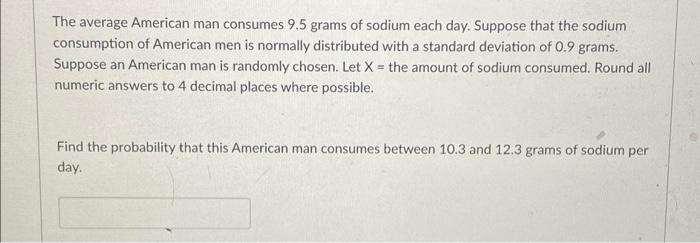Home / Expert Answers / Statistics and Probability / the-average-american-man-consumes-9-5-grams-of-sodium-each-day-suppose-that-the-sodium-consumption-pa588

# (Solved): The average American man consumes 9.5 grams of sodium each day. Suppose that the sodium consumption ...The average American man consumes 9.5 grams of sodium each day. Suppose that the sodium consumption of American men is normally distributed with a standard deviation of 0.9 grams. Suppose an American man is randomly chosen. Let the amount of sodium consumed. Round all numeric answers to 4 decimal places where possible. Find the probability that this American man consumes between 10.3 and 12.3 grams of sodium per day.

We have an Answer from Expert